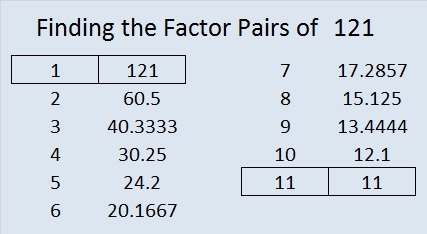# 121 and Level 6

121 is not a clue in today’s puzzle. However, when 121 is a clue in a FIND THE FACTORS 1 – 12 puzzle, place 11 in the corresponding cells in both the factor row and the factor column.This week’s puzzles and last week’s factors: 10 Factors 2014-05-12

Here’s more about the number 121:

• 121 is a composite number.
• Prime factorization: 121 = 11 × 11 which can be written 121 = 11²
• The exponent in the prime factorization is 2. Adding one we get (2 + 1) = 3. Therefore 121 has exactly 3 factors.
• Factors of 121: 1, 11, 121
• Factor pairs: 121 = 1 × 121 or 11 × 11
• 121 is a perfect square. √121 = 11121 = 11². Those digits 1, 1, 2 make 121 the second Friedman number because they can be arranged as a mathematical expression that equals a number that uses those same digits.

121 is not only a perfect square but it is also a star! In fact, games of Chinese checkers will be based on that perfect star.

Since 121 is the square of 11, the fifth prime number, it is only the fifth number to have exactly 3 factors.

121 is the sum of the three prime numbers from 37 to 43.

121 looks interesting when it is written in some other bases:
It’s repdigit 11111 in BASE 3 because 3⁴ + 3³ + 3² + 3¹ + 3⁰ = 121
and it’s 321 in BASE 6.

Not only is it a palindrome in base 10, but
it’s 232 in BASE 7, and
it’s 171 in BASE 8

It can also look pretty square depending on the base used:
It’s 441 in BASE 5,
144 in BASE 9,
121 in BASE 10 (of course),
100 in BASE 11,
81 in BASE 15, and
49 in BASE 28

121 is a pretty remarkable number!

If you got stuck solving the puzzle above, here’s one logical way to find the solution:## One thought on “121 and Level 6”

This site uses Akismet to reduce spam. Learn how your comment data is processed.